# Solving Most Systems of Random Quadratic Equations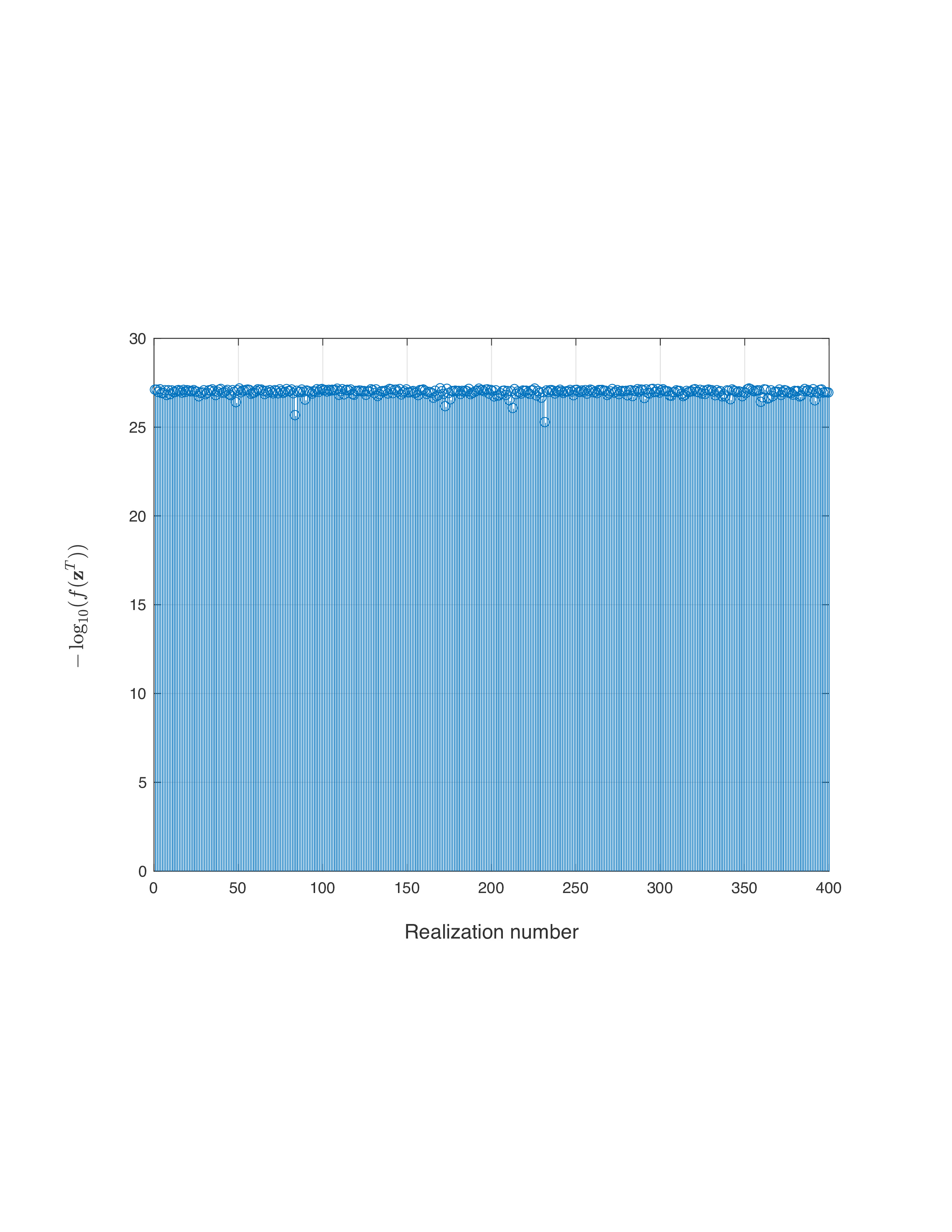To show the power of RAF in the high-dimensional regime, the amplitude-based least-squares loss function value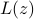evaluated at the returned estimate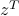for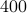MC realizations is plotted (in negative logarithmic scale) in figure on the left, where the number of simulated noiseless measurements was set to be the information-theoretic limit, namely,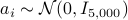, and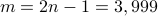for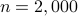. It is self-evident that our proposed RAF approach returns a solution of function value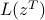smaller than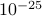in allindependent realizations even at this challenging information-theoretic limit condition. To the best of our knowledge, RAF is the first algorithm that empirically reconstructs any high-dimensional (say e.g.,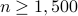) signals exactly from an emph{optimal number} of random quadratic equations, which also provides a positive answer to the question posed easier in the Introduction.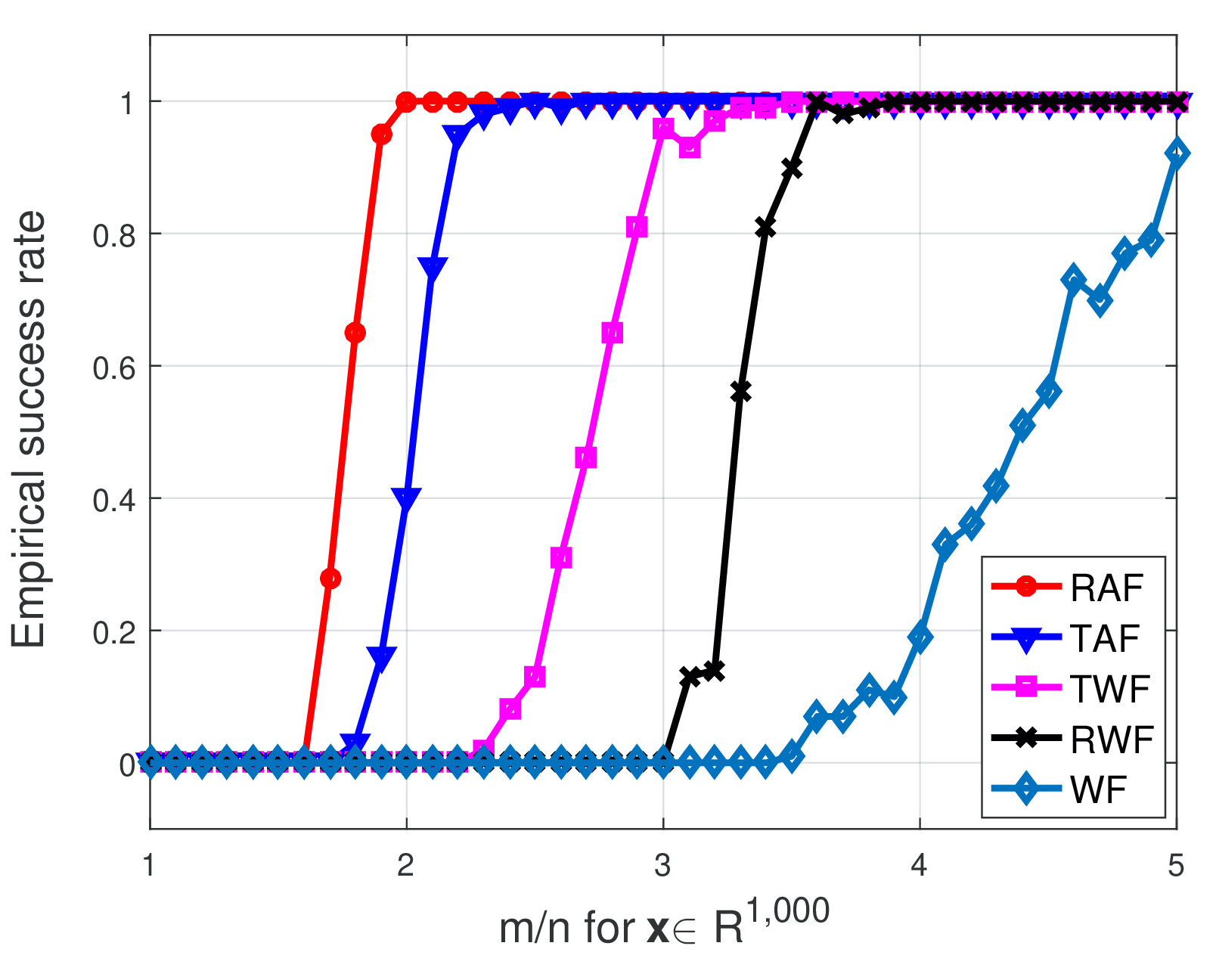The left figure compares the empirical success rate of five schemes with the signal dimension being fixed at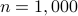while the ratio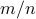increasing by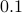fromto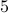. As clearly depicted by the plots, our RAF (the red plot) enjoys markedly improved performance over its competing alternatives. Moreover, it also achieves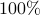perfect signal recovery as soon as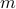is about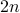, where the others do not work (well).
Paper details

### Solving Most Systems of Random Quadratic Equations (Preprint),(Poster)

Authors: G. Wang, G. B. Giannakis, Y. Saad, and J. Chen

This paper deals with finding an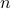-dimensional solution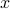to a system of quadratic equations of the form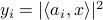for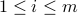, which is also known as phase retrieval and is NP-hard in general. We put forth a novel procedure for minimizing the amplitude-based least-squares empirical loss, that starts with a weighted maximal correlation initialization obtainable with a few power or Lanczos iterations, followed by successive refinements based upon a sequence of iteratively reweighted (generalized) gradient iterations. The two (both the initialization and gradient flow) stages distinguish themselves from prior contributions by the inclusion of a fresh (re)weighting regularization technique. The overall algorithm is conceptually simple, numerically scalable, and easy-to-implement. For certain random measurement models, the novel procedure is shown capable of finding the true solutionin time proportional to reading the data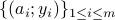. This holds with high probability and without extra assumption on the signalto be recovered, provided that the numberof equations is some constant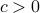times the numberof unknowns in the signal vector, namely,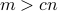. Empirically, the upshots of this contribution are: i) (almost)perfect signal recovery in the high-dimensional (say e.g.,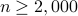) regime given only an emph{information-theoretic limit} number of noiseless equations, namely,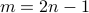in the real-valued Gaussian case; and, ii) (nearly) optimal statistical accuracy in the presence of additive noise of bounded support. Finally, substantial numerical tests using both synthetic data and real images corroborate markedly improved signal recovery performance and computational efficiency of our novel procedure relative to state-of-the-art approaches.